SSC BOARD PAPERS IMPORTANT TOPICS COVERED FOR BOARD EXAM 2024

### Practice Set 4.3 Financial Planning Class 10th Mathematics Part 1 MHB Solution

Practice Set 4.3

1. Complete the following table by writing suitable numbers and words.…
2. Amol purchased 50 shares of Face Value Rs. 100 when the Market value of the share was…
3. Joseph purchased following shares, Find his total investment. Company A : 200 shares,…
4. Smt. Deshpande purchased shares of FV Rs. 5 at a premium of Rs. 20. How many shares…
5. Shri Shantilal has purchased 150 shares of FV Rs. 100, for MV of Rs. 120. Company has…
6. If the face value of both the shares is same, then which investment out of the…
###### Practice Set 4.3
Question 1.

Complete the following table by writing suitable numbers and words.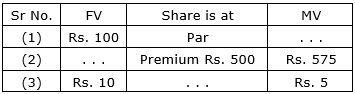(1) We know that if MV = FV, then the share is at par.

Here, FV = Rs. 100

∴ MV = Rs. 100

(2) We know that if MV > FV, then the share is at premium.

∴ FV = MV – Premium

⇒ FV = 575 – 500 = Rs. 75

(3) We know that FV = Rs. 10 and MV = Rs.3

⇒ FV – MV = 10 – 5 = Rs. 5

We know that if MV < FV, then the share is at discount.

∴ The given share is at discount at Rs. 5.

Question 2.

Amol purchased 50 shares of Face Value Rs. 100 when the Market value of the share was Rs. 80. Company had given 20% dividend. Find the rate of return on investment.

Given FV = Rs. 100; MV = Rs. 80; D = 20%

On investment of Rs. 80, Amol got Rs. 20.

Let rate of return be x%.

∴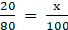⇒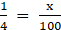∴ x =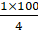⇒ x = 25% (Rate of Return)

Question 3.

Joseph purchased following shares, Find his total investment.

Company A : 200 shares, FV = Rs. 2 Premium = Rs. 18.

Company B : 45 shares, MV = Rs. 500

Company C : 1 share, MV = Rs. 10,540.

Company A: Premium = Rs. 18

We know that if MV > FV, then the share is at premium.

∴ MV = FV + Premium

⇒ MV = 2 + 18 = Rs.20

We know that investment = number of shares × MV

∴ Investment in company A = 200 × 20

= Rs. 4000

Company B:

∴ Investment in company B = 45 × 500

= Rs. 22, 500

Company C:

∴ Investment in company C = 1 × 10, 540

= Rs. 10, 540

Joseph has invested 4000 + 22, 500 + 10, 540 = Rs. 37, 040

Question 4.

Smt. Deshpande purchased shares of FV Rs. 5 at a premium of Rs. 20. How many shares will she get for Rs. 20,000?

We know that if MV > FV, then the share is at premium.

∴ MV = FV + Premium

⇒ MV = 5 + 20

= Rs. 25

We know that investment = number of shares × MV

Given, investment = Rs. 20, 000

∴ Number of shares =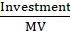⇒ Number of shares =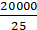= 800

Question 5.

Shri Shantilal has purchased 150 shares of FV Rs. 100, for MV of Rs. 120. Company has paid dividend at 7%. Find the rate of return on his investment.

Given, number of shares = 150

FV = Rs. 100

MV = Rs. 120

Dividend = 7%

We know that investment = number of shares × MV

∴ Investment = 150 × 120

= Rs. 18, 000

We know that dividend per share =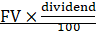∴ Dividend per share =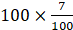= Rs. 7

∴ Total dividend received = 150 × 7 = Rs. 1050

We know that rate of return =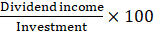∴ Rate of return =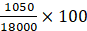= 5.83%

Question 6.

If the face value of both the shares is same, then which investment out of the following is more profitable?

Company A : dividend 16%, MV = Rs. 80, Company B : dividend 20%, MV = Rs. 120.

Let FV = Rs. 100

Company A:

We know that dividend per share =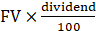∴ Dividend per share =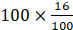= Rs. 16

Income on Rs. 1 =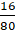= Rs. 0.20

Company B:

We know that dividend per share =∴ Dividend per share =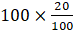= Rs. 20

Income on Rs. 1 =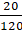= Rs. 0.16

∴ Investment of company A is more profitable than company B.